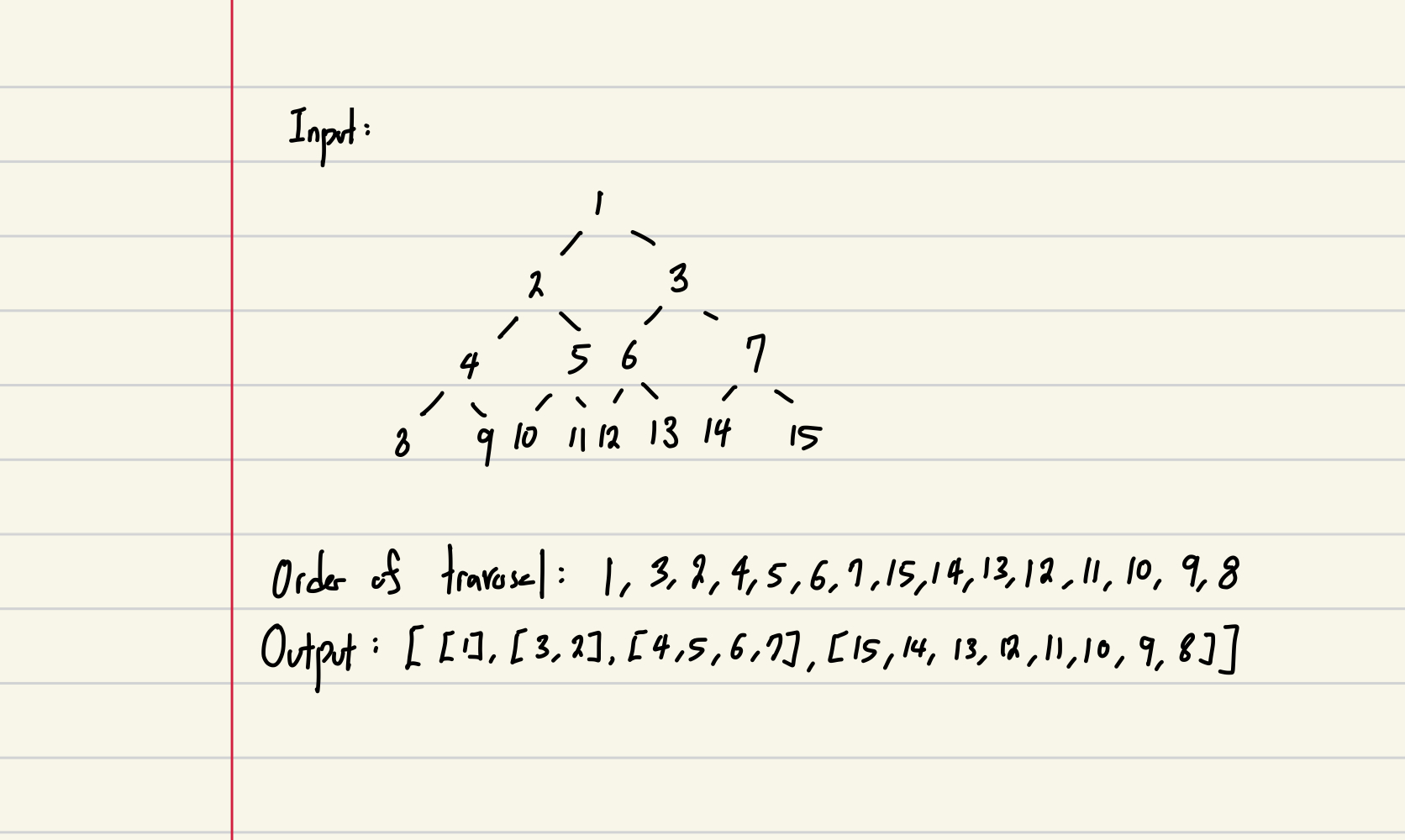# [LeetCode] #103: Binary Tree Zigzag Level Order Traversal

choikh0423·2022년 5월 11일
0

## LeetCode

목록 보기
9/11## Problem:

Given the root of a binary tree, return the zigzag level order traversal of its nodes' values. (i.e., from left to right, then right to left for the next level and alternate between).

## Process:

Objective: Returning the values of nodes in the order of zigzag level order traversal.

Constraints:
1. The number of nodes in the tree is in the range [0, 2000].
2. -100 <= Node.val <= 100

Edge Cases:
1) When the tree is empty
2) When the tree only has one node
3) When the tree has multiple nodes in multiple depths

## Brainstorm:

What is a Zigzag Level Order Traversal?
Zigzag level order traversal reverses the direction of traversal at each depth.

Here is an example input and output of Zigzag Level Order Traversal.Two Key Elements of Zigzag Level Order Traversal
1. It is Level Order Traversal. We have to traverse the nodes on one depth first in order to get to the next depth.
2. The direction of traversal will reverse for each depth. Even Levels will go from left to right and odds will go in reverse.

We Can Say That:
At Depth 0: the direction of traversal is left to right
At Depth 1: the direction of traversal is right to left
At Depth 2: the direction of traversal is left to right
and so on.

When the direction goes from left to right:
nodes_to_visit: [leftmost node in tree is in the leftmost side of list]

1. We will be popping nodes from right to left from nodes_to_visit list. The nodes_to_visit list will have the leftmost node at the rightmost side of the list. Since we want to traverse from the leftmost node of the tree, we pop from the right of the list.
2. We will be appending the children to the left since we don't want to interfere traversing the previous level which is popping from right.
3. We will be visiting from left to right child in that order. Since we are visiting the left node first, we want to make the children nodes to be in consecutive order. This creates nodes_to_visit to have the rightmost node on the leftmost side of the list.

When the direction goes from right to left:
nodes_to_visit: [leftmost node in tree is in the leftmost side of list]

1. We will be popping nodes from left to right from nodes_to_visit list. The nodes_to_visit list will have the rightmost node of the tree depth at the leftmost side of the list. Since we want to traverse from the rightmost node of the tree, we pop from the left of the list.
2. We will be appending the children to the right since we don't want to interfere traversing the previous level which is popping from left.
3. We will be visiting from right to left child in that order. Since we are visiting the right node first, we want to make the children nodes to b ein consecutive order by creating nodes_to_visit to be a list with leftmost node in the rightmost side of the list.

When we are done with each depth:
1. Update the number of nodes in each depth.
2. Append the values list for that depth to the final list of values
3. Re-initialize the values list of depth to be an empty list. Also, update the number of nodes of that level.

It would be easy to say: Why not reverse the list when we append to the result list
Reversing the list will cost around (n/2) assuming that half of the nodes will be reversed. Therefore, it will cause our time complexity to be O(n^2).

## Solution:

### Attempt #1: Using the method described above

• Time Complexity: O(n) where n is the number of nodes in the tree
• Space Complexity: O(n) ~ O(3n)

How it works:
Read the description above in the brainstorm part of this post!

from collections import dequeue

def zigzagLevelOrder(self, root: Optional[TreeNode]) -> List[List[int]]:
nodes_to_visit = deque()
final_value_list = []
depth_value_list = []
node = root

nodes_in_level = 1

# Will append children from Right to Left if 1
traversal_direction_LR = 1

while node:
depth_value_list.append(node.val)

# Adding children of Treenode to nodes_to_visit
if traversal_direction_LR == 1:
if node.left:
nodes_to_visit.appendleft(node.left)
if node.right:
nodes_to_visit.appendleft(node.right)
else:
if node.right:
nodes_to_visit.append(node.right)
if node.left:
nodes_to_visit.append(node.left)

# Updating nodes_in_level
nodes_in_level -= 1

# Updating nodes_in_level when done with one depth
if nodes_in_level == 0:
final_value_list.append(depth_value_list)
depth_value_list = []
nodes_in_level = len(nodes_to_visit)
traversal_direction_LR *= -1

# Updating node
if nodes_to_visit:
if traversal_direction_LR == 1:
node = nodes_to_visit.pop()
else:
node = nodes_to_visit.popleft()
else:
node = None

return final_value_list

Limitations:

1. It takes up quite a bit of space. I could probably get this down to a tight O(n) space wise.

LeetCode Question #103